# Kerala Syllabus 9th Standard Maths Solutions Chapter 2 Decimal Forms

## Kerala State Syllabus 9th Standard Maths Solutions Chapter 2 Decimal Forms

### Kerala Syllabus 9th Standard Maths Decimal Forms Text Book Questions and Answers

Textbook Page No. 26

Decimal Forms Class 9 Kerala Syllabus Question 1.
Write the fractions below in decimal form:

1. $$\frac{3}{20}$$
2. $$\frac{3}{40}$$
3. $$\frac{13}{40}$$
4. $$\frac{7}{80}$$
5. $$\frac{5}{16}$$

1. $$\frac{3}{20}$$
= $$\frac{3×5}{20×5}$$ = $$\frac{15}{100}$$ = 0.15

2. 40 = 2 x 2 x (2 x 5)
Multiplied by 5 x 5
(2 x 5) x (2 x 5) x (2 x 5) = 1000
it is a multiple of 10.
$$\frac{3}{10}$$ = $$\frac{3x5x5}{40x4x5x}$$ = $$\frac{75}{1000}$$ = 0.75

3. 40 = 2 x 2 x (2 x 5)
Multiplied by 5 x 5
(2 x 5) x (2 x 5) x (2 x 5) = 1000
It is a multiple of 10.
$$\frac{13}{40}$$ = $$\frac{13x5x5}{20}$$ = $$\frac{325}{1000}$$ = 0.325

4. 80 = 2 x 2 x 2 x (2 x 5)
Multiplied by 5 x 5 x 5
It is a multiple of 10.
(2 x 5) x (2 x 5) x (2 x 5) x (2 x 5) = 1000
$$\frac{7}{80}$$ = $$\frac{7x5x5x5}{80x5x5x5}$$ = $$\frac{875}{10000}$$ = 0.0875

5. 16 = 2 x 2 x 2 x 2
Multiplied by 5 x 5 x 5 x 5 x 5
(2 x 5) x (2 x 5) x (2 x 5) x (2 x 5) = 1000
It is a multiple of 10.
$$\frac{5}{16}$$ = $$\frac{5x5x5x5x5}{16x5x5x5x5}$$ = $$\frac{3125}{10000}$$ = 0.3125

Kerala Syllabus 9th Standard Maths Chapter 2 Question 2.
Find the decimal form of the sums below:

1. $$\frac{1}{80}$$ + $$\frac{1}{25}$$ + $$\frac{1}{125}$$
2. $$\frac{1}{5}$$ + $$\frac { 1 }{ { 5 }^{ 2 } }$$ + $$\frac { 1 }{ { 5 }^{ 3 } }$$ + $$\frac { 1 }{ { 5 }^{ 4 } }$$
3. $$\frac{1}{2}$$ + $$\frac { 1 }{ { 2 }^{ 2 } }$$ + $$\frac { 1 }{ { 2 }^{ 3 } }$$

1. $$\frac{1}{80}$$ + $$\frac{1}{25}$$ + $$\frac{1}{125}$$
Denominators of all the fractions should be 125
$$\frac{1×25}{5×25}$$ + $$\frac{1×5}{25×5}$$ + $$\frac{1×1}{125×1}$$
= $$\frac{25}{125}$$ + $$\frac{5}{125}$$ + $$\frac{1}{125}$$ = $$\frac{31}{125}$$
125 = 5 x 5 x 5
Multiplied by 2 x 2 x 2
(5 x 2) x (5 x 2) x (5 x 2) = 1000
It is a power of 10
$$\frac{31}{125}$$ = $$\frac{31x2x2x2}{125x2x2x2}$$ = $$\frac{248}{1000}$$ = 0.248

2. $$\frac{1}{5}$$ + $$\frac { 1 }{ { 5 }^{ 2 } }$$ + $$\frac { 1 }{ { 5 }^{ 3 } }$$ + $$\frac { 1 }{ { 5 }^{ 4 } }$$
Denominators of all the fractions should be 54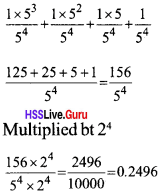3. $$\frac{1}{2}$$ + $$\frac { 1 }{ { 2 }^{ 2 } }$$ + $$\frac { 1 }{ { 2 }^{ 3 } }$$
Denominators of all the fractions should be 23Hss Live Guru 9th Maths Kerala Syllabus Question 3.
A two – digit number divided by an other two-digit number gives 5.875. What are the numbers?
5875 = $$\frac{5875}{1000}$$
5875 = 5 x 5 x 5 x 47
1000 = 5 x 5 x 5 x 85.875 = $$\frac{5875}{1000}$$ = $$\frac{5x5x5x47}{5x5x5x8}$$ = $$\frac{47}{8}$$
But 8 is not a two digit number. So we multiplied both numerator and denominator by 2, we get
$$\frac{47}{8}$$ = $$\frac{94}{16}$$
5.875 = $$\frac{94}{16}$$

Textbook Page No. 30

Kerala Syllabus 9th Standard Maths Notes Question 1.
For each of the fractions below, find fractions with denominators powers of 10 gefting chaser and closer to it and hence write its decimal form:

1. $$\frac{2}{3}$$
2. $$\frac{5}{6}$$
3. $$\frac{1}{9}$$

1. $$\frac{2}{3}$$ = $$\frac{2×10}{3×10}$$ = $$\frac{2}{10}$$ x $$\frac{10}{3}$$ = $$\frac{2}{10}$$ x (3 + $$\frac{1}{3}$$)The fraction $$\frac{6}{10}$$, $$\frac{66}{100}$$, $$\frac{666}{1000}$$, ……… and so on get closer and closer to $$\frac{2}{3}$$.
i.e., 0.6, 0.66, 0.666, …………..
$$\frac{1}{2}$$ = 0.666

2. $$\frac{5}{6}$$ = $$\frac{5×10}{6×10}$$ = $$\frac{5}{10}$$ x $$\frac{10}{6}$$ = $$\frac{5}{10}$$ x (1 + $$\frac{4}{6}$$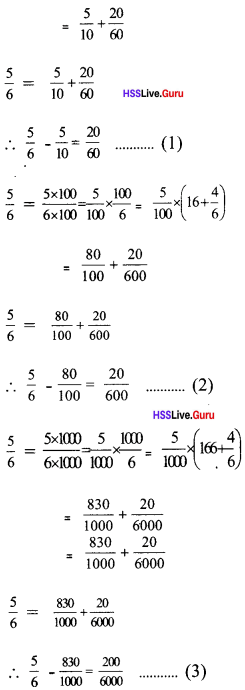The fractions $$\frac{80}{100}$$, $$\frac{830}{1000}$$, $$\frac{8330}{5}$$, ………. and so on get closer and closer to $$\frac{5}{6}$$.
∴ $$\frac{5}{6}$$ = 0.8333………

3. $$\frac{1}{9}$$ = $$\frac{1×10}{9×10}$$ = $$\frac{1}{10}$$ x $$\frac{10}{9}$$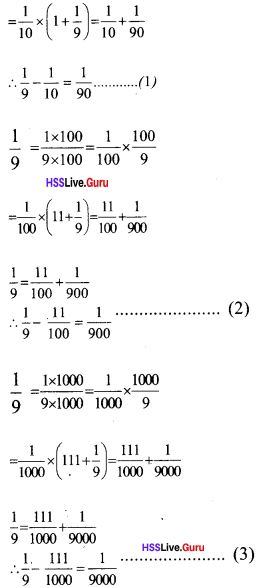The fractions $$\frac{1}{10}$$, $$\frac{11}{100}$$, $$\frac{111}{1000}$$, ………… and so on get closer and closer to $$\frac{1}{9}$$.
∴ $$\frac{1}{9}$$ = 0.1111 ……

Hsslive Maths Class 9 Kerala Syllabus Question 2.

1. Using algebra, explain why $$\frac{1}{10}$$, $$\frac{11}{100}$$, $$\frac{1}{1000}$$, ………… of any number get closer and closer to $$\frac{1}{9}$$ of that number.
2. Use the general principle got above to single-digit numbers to find the decimal forms of $$\frac{2}{9}$$, $$\frac{4}{9}$$, $$\frac{5}{9}$$, $$\frac{7}{9}$$, $$\frac{8}{9}$$.
3. What can we say in general about decimal forms with a single digit repeating?

1. Let x be the numberThe numbers $$\frac{x}{10}$$, $$\frac{11x}{100}$$, $$\frac{111x}{1000}$$, ……… comes closer and closer to $$\frac{x}{9}$$.
So the fraction $$\frac{1}{10}$$, $$\frac{11}{100}$$, $$\frac{111}{1000}$$, ……. comes closer and closer to $$\frac{1}{9}$$.

2. a.
$$\frac{1}{10}$$ part of a number comes closer to its $$\frac{1}{9}$$b. $$\frac{1}{10}$$ part of a number comes closer to its $$\frac{1}{9}$$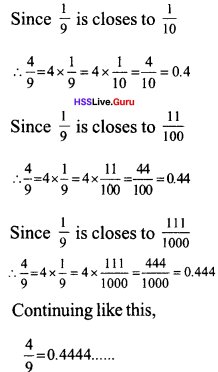c. $$\frac{1}{10}$$ part of a number comes closer to its $$\frac{1}{9}$$d. Since $$\frac{1}{9}$$ is closes to $$\frac{1}{10}$$e. Since $$\frac{1}{9}$$ is closes to $$\frac{1}{10}$$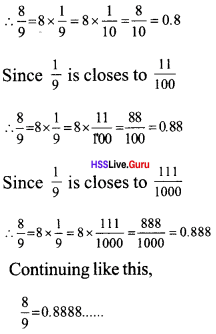3. The denominator of a fraction is 9 or multiple of 9 then its decimal froms is always a single digit repetition of their own numerator.

Hsslive Guru 9th Maths Kerala Syllabus Question 3.

1. Find the decimal form of $$\frac{1}{11}$$.
2. Find the decimal forms of $$\frac{2}{11}$$, $$\frac{3}{11}$$
3. What is the decimal form of $$\frac{10}{11}$$?

1. $$\frac{1}{11}$$ = $$\frac{1×10}{11×10}$$ = $$\frac{1}{10}$$ x $$\frac{10}{11}$$Continuing like this,
= 0.090909

2. a. $$\frac{2}{11}$$ = $$\frac{2×10}{11×10}$$ + $$\frac{2}{10}$$ x $$\frac{10}{11}$$Continuing like this,
$$\frac{2}{11}$$ = 0.18181818 …….

b. $$\frac{3}{11}$$ = $$\frac{3×10}{11×10}$$ = $$\frac{3}{10}$$ x $$\frac{10}{11}$$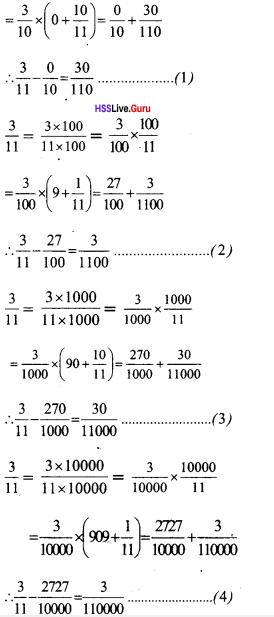Continuing like this
$$\frac{3}{11}$$ = 0.272727…..

3. $$\frac{10}{11}$$ = $$\frac{10×10}{11×10}$$ = $$\frac{10}{10}$$ x $$\frac{10}{11}$$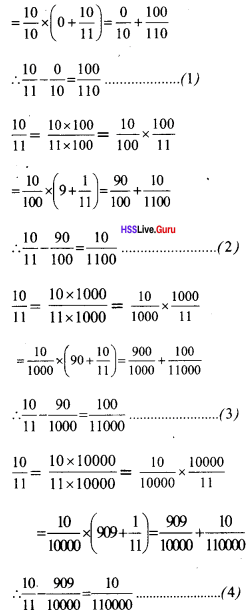Continuing like this
$$\frac{10}{11}$$ = 0.90909……….

Hss Live Guru 9 Maths Kerala Syllabus Question 4.
Write the results of the operations below as decimals:

1. 0.111…. + 0.222 …….
2. 0.333…. + 0.777 …..
3. 0.333…. x 0.666…
4. (0.333….)2
5. $$\sqrt { 0.444…… }$$

1. 0.111 = $$\frac{1}{9}$$
0.222…. = $$\frac{2}{9}$$
0.111 …. + 0.2222 …….. = $$\frac{1}{9}$$ + $$\frac{2}{9}$$ = $$\frac{3}{9}$$
= 0.3333

2. 0.3333 = $$\frac{3}{9}$$
0.7777…. = $$\frac{7}{9}$$
0.3333 ……. + 0.7777…. = $$\frac{1}{9}$$ = $$\frac{2}{9}$$ = $$\frac{3}{9}$$
= 1.1111……….

3. 0.3333 = $$\frac{3}{9}$$
0.6666…. = $$\frac{6}{9}$$
0.3333….. x 0.6666…… = $$\frac{3}{9}$$ x $$\frac{6}{9}$$
= $$\frac{18}{81}$$ = $$\frac{2}{9}$$ = 0.2222……..

4. 0.3333 …….. = $$\frac{3}{9}$$
(0.3333)2 ….. = ($$\frac{3}{9}$$)2
= $$\frac{3×3}{9×9}$$ = $$\frac{1}{9}$$ = 0.1111…………

5. 0.4444 ….. = $$\frac{4}{9}$$### Kerala Syllabus 9th Standard Maths Decimal Forms Exam Oriented Question and Answers

Question 1.
Write the deciamal form of $$\frac{1}{6}$$
$$\frac{1}{6}$$ = 0.1666…….

Question 2.
Write in deciamals

1. $$\frac{1}{9}$$
2. $$\frac{2}{9}$$
3. $$\frac{1}{7}$$
4. $$\frac{1}{11}$$
5. $$\frac{2}{11}$$
6. $$\frac{1}{12}$$

1. 0.111……
2. 0.222………
3. 0.14285…..
4. 0.090909…..
5. 0.181818….
6. 0.08333……

Question 3.
Find the fraction of denominator is a power of 10 equal to each of the fractions below, and then write their decimal forms:
i. $$\frac{1}{50}$$
ii. $$\frac{3}{40}$$
iii.$$\frac{5}{16}$$
iv. $$\frac{12}{625}$$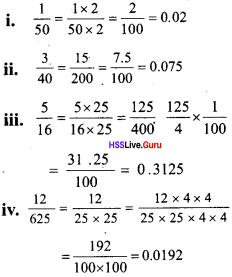Question 4.
Find the fraction of denominator is a power of 10 getting closer and closer to each of the fractions be low, and then write their decimal forms.

1. $$\frac{5}{6}$$
2. $$\frac{3}{11}$$
3. $$\frac{23}{11}$$
4. $$\frac{1}{13}$$

1. $$\frac{5}{6}$$ = $$\frac{5}{10}$$ x $$\frac{10}{6}$$ = $$\frac{1}{10}$$($$\frac{50}{6}$$)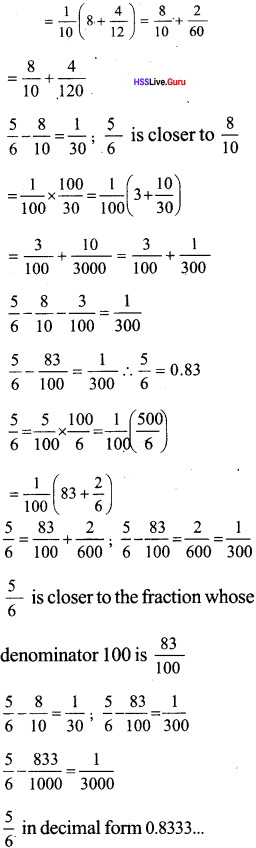2. $$\frac{3}{11}$$ = $$\frac{3}{100}$$ x $$\frac{100}{11}$$ = $$\frac{3}{100}$$(9 + $$\frac{1}{11}$$)3. $$\frac{23}{11}$$ = $$\frac{23}{100}$$ x $$\frac{100}{11}$$4. $$\frac{1}{13}$$ = $$\frac{1}{100}$$ x $$\frac{100}{13}$$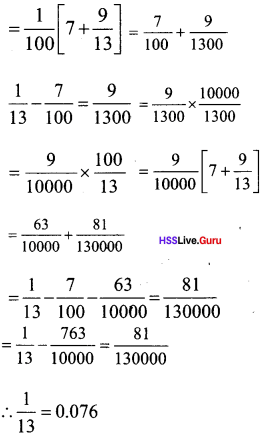Question 5.

1. Explain using algebra, that the fractions $$\frac{1}{10}$$, $$\frac{11}{100}$$, $$\frac{111}{1000}$$… gets closer and closer to $$\frac{1}{9}$$
2. Using the general principle above on single digit numbers, find the decimal forms of $$\frac{2}{9}$$, $$\frac{4}{9}$$, $$\frac{5}{9}$$, $$\frac{7}{9}$$, $$\frac{8}{9}$$ (Why $$\frac{3}{9}$$ and $$\frac{6}{9}$$ left out in this?)
3. What can we say in general about those decimal forms In which a single digit repeats?

1. Let x be the number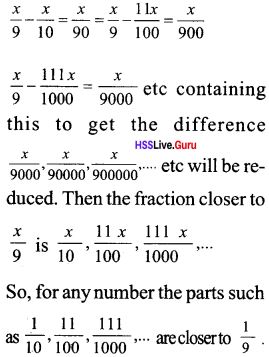2. $$\frac{2}{9}$$ – $$\frac{2}{10}$$ = $$\frac{2}{90}$$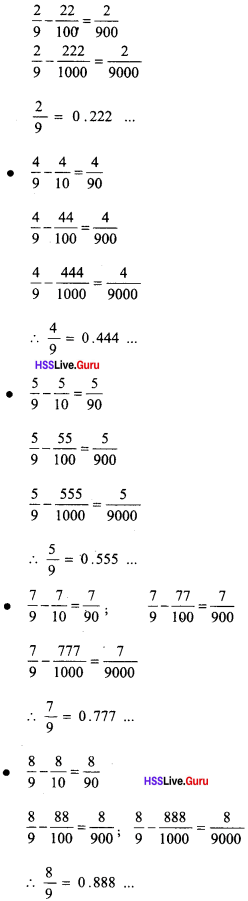($$\frac{3}{9}$$, $$\frac{6}{9}$$ These fractions having common factor in the numerator and the denominator)

3. Repeated deciamals.

Question 6.

1. Find the decimal form of $$\frac{1}{4}$$.
2. Write the decimal form of $$\frac{7}{10}$$ + $$\frac{3}{100}$$ + $$\frac{4}{1000}$$.

1. $$\frac{1}{4}$$ = 0.25

2. $$\frac{7}{10}$$ = 0.7
$$\frac{3}{100}$$ = 0.03
$$\frac{4}{1000}$$ = 0.004
$$\frac{7}{10}$$ + $$\frac{3}{100}$$ + $$\frac{4}{1000}$$
= 0.7 + 0.03 + 0.004
= 0.734

Question 7.

1. Write the decimal forms of $$\frac{1}{3}$$ and $$\frac{1}{9}$$.
2. What is the decimal form of (0.3333…..)2?

1. $$\frac{1}{3}$$ = 0.3333…..
$$\frac{1}{9}$$ = 0.1111……

2. (0.3333…..)2 = ($$\frac{1}{3}$$)2 = $$\frac{1}{9}$$ = 0.1111….

Question 8.
Write the decimal forms of $$\frac{3}{25}$$ and $$\frac{1}{8}$$.a. Write the decimal form of the fractions and $$\frac{1}{2}$$ and $$\frac{2}{5}$$.
b. 1f f is a fraction between and $$\frac{2}{5}$$ What is k?
c. Write the decimal form of the fraction $$\frac{4}{k}$$.If $$\frac{4}{k}$$ is between $$\frac{1}{2}$$ and $$\frac{2}{5}$$, then $$\frac{4}{k}$$ is between $$\frac{4}{8}$$ and $$\frac{4}{10}$$. Then the number is $$\frac{4}{9}$$.
c. $$\frac{4}{9}$$ = 0.4444…….